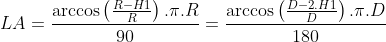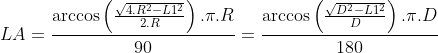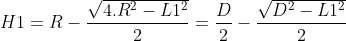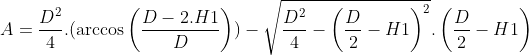# Circle segment calculator, formulas, practical use.

## Calculator for a circle segment calculations

The circle segment calculator is designed for calculations radius and diameter of a circle segmentcircumference and area of a circle segment, chord and arc length of a circle segment and height of a circle segmentFor successful calculations, you need to know and enter into the calculator radius or diameter and at least one of the other valuesOr chord length and height of a circle segment. Then press send or enter to run calculation

## Parameters sketch:## Calculator form:

﻿
Unit system:
•

•

•

•

•

•

•

## Variables and constants explanation:

d – diameter
C – circumference
A – area
LA – arc length
π– 3,1415926535
H1– section height
L1 – chord length
– unspecified values
specified values
calculated values

## Circle arc length calculation formula 1:## Circle arc length calculation formula 2:## Circle chord length calculation formula:## Circle segment height calculation formula:## Circle segment area calculation formula 1:## Circle segment area calculation formula 2:## Circle segment circumference calculation formula:## Calculator use:

Calculations of circle segment for:

• technical calculation of D-shaped trimmed shaft in the engineering industry
• the cross-sectional area of a truncated cylinder, truncated tree trunk, pipe, tube, hose, rod, tunnel, pillar
• school calculations of a truncated cylinder